Independent Component Analysis of Evoked Potentials in EEG

Michael Vinther, s973971   mv@logicnet.dk

Ørsted, DTU

December 8th, 2002

Supervisor: Kaj-Åge Henneberg# 2 Introduction

EEG obtained from scalp electrodes is a sum of the large number of brain cell (neuron) potentials. When examining the EEG, the issue of interest is often not the potentials on the scalp, but the potentials in the sources inside the brain. Direct measurements from the different centers in the brain require placing electrodes inside the head, which means surgery. This is undesirable primarily because it causes pain and risk for the subject. A better solution would be if it were possible to calculate the desired signals from the EEG obtained on the scalp.

The obtained signals are weighed sums of the cell potentials, where the weights depend on the signal path from the neurons to the electrodes. Because the same potential is recorded from more than one electrode, the signals from the electrodes are highly correlated. If the weights were known, the potentials in the sources could be computed from a sufficient number of electrode signals. Independent component analysis (ICA), sometimes referred to as blind signal separation or blind source separation, is a mathematical tool that can help solving the problem.

The algorithms for ICA were developed in the last ten years, so it is quite a new field of research. Pope and Bogner (1996 I & II) have a review of the early literature in the field. In the following description, the number of sources separated is the same as the number of sensors (here electrodes), and no time delay is assumed. It is primarily based on Hyvärinen and Oja (1999), which gives a good introduction to the method. Højen-Sørensen et al. (2001) describes an approach capable of separating more sources than there are sensors. Time delayed and/or convolved signals are treated in Pope and Bogner (1996 II).

Separation of sources in evoked potentials (EPs) and reduction of artifacts in EEG recordings are the areas that ICA will be applied to in this report. ICA of EPs demonstrates the methods ability to separate processes. Evoked potentials are extracted from many recordings of the same epoch of response. A blink of an eye or just eye movement will produce artifacts in the EEG obtained at the electrodes and may render that epoch unusable. If a limited number of epochs are available, or it is not possible to instruct the subject not to move during the recording (this will be the case with animal tests), such artifacts can be a problem. Jung et al. (1998) uses ICA for reducing artifacts in EEG, which could increase the number of usable epochs. That method will be described and tested here, and the examples clearly show the strength of the technique.

EEG recordings with responses to click sounds from a group of test subjects were used as example data. The stimulus was presented to each subject 120 times, and epochs where noise forced the A/D converter to saturation were removed. The sampling was done at 1 kHz. This data set was made available to me by Sidse Arnfred, dept. of psychiatry, Bispebjerg Hospital.

Section 3  following this introduction defines the ICA problem, demonstrates how it can be solved and finally gives a complete ICA algorithm. An implementation of the algorithm is included in appendix. In section 4  it is explained how ICA can be applied to EEG data, and examples of the two applications are given.

# 3 Independent component analysis

The “standard” example of independent component analysis is the so-called cocktail-party problem: In a room where several people are speaking at the same time, identify what each person is saying by listening to the mixture of voices. Most people are able to do that by just listening and focusing on a specific speaker.

## 3.1 Definition

In general, it is the problem of separating independent sources from mixtures obtained at different sensors. Different versions of the algorithm with different properties are described in the literature as mentioned in the introduction, but the method used here work under the following assumptions:

1.       Sources are statistically independent (independent components)

2.      At most one of the sources can have a Gaussian distribution

3.      Signals at the sensors are different linear combinations of the sources

4.      No time delay from sources to sensors

5.      Same number of sources and sensors

Both the sources and the weights in the linear combinations can be estimated with no other knowledge of the system. In the cocktail-party problem, assumption 4 is actually not satisfied because of the limited speed of sound, so the mixture of sounds recorded in an instant at each microphone might not be produced simultaneously. The same is true for many other real-world problems: The assumptions are not completely satisfied, but ICA is often able to give a good estimate.

With two sources and two sensors, it can be formulated as follows: x1(t) and x2(t) are linear combinations of the sources s1(t) and s2(t).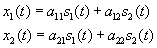(1)

Find a11, a12, a21, a22, s1(t) and s2(t) for known x1(t) and x2(t). In the following, matrix-vector notation will be used: Vectors are written in bold (s) and matrices in bold capitals (A). Elements in vectors/matrices are denoted as scalars with index (a11, si). The linear combination of sources is now

x = As.                                                                      (2)

The solution is found with the following limitations:

1.      The energy of the sources cannot be determined because a scalar multiplier in si results in the same x as a scaling of the i’th column in A.

2.      Sign of the sources cannot be determined for the same reason.

3.      The order of the sources cannot be determined, because swapping two sources results in the same x as swapping the corresponding columns in A.

For many problems this is not significant, or can be determined posterior to the ICA because of other knowledge about the sources.

Why and how it is possible to find A and s will be illustrated with two example problems. Figure 1 show 1000 samples from two systems of two sources. The sources in the left plot are random with a uniform distribution, and to the right are a sine and a triangular wave with different period to ensure independence. All sources have unit variance.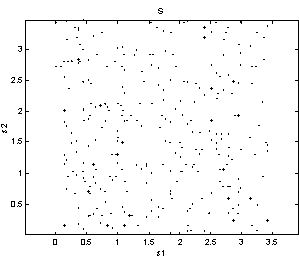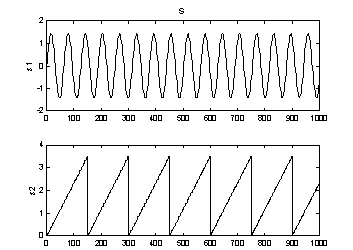Figure 1: Two problems with two sources of unit variance.

In Figure 2 the sources have been linearly transformed with the matrix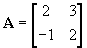(3)

to produce the x signals, simulating recordings of mixed sources. Just by looking at the (x1,x2) plot, one would still be able to draw the axes of the sources, because the shape of the original distributions is still recognizable. And as the axes are the vectors forming the rows in A, this is actually solving the problem! When the axes and thereby A are found, s can be computed as

s = A-1x.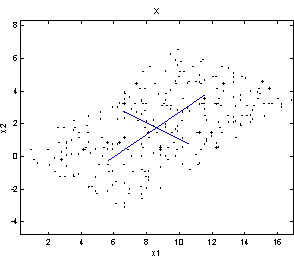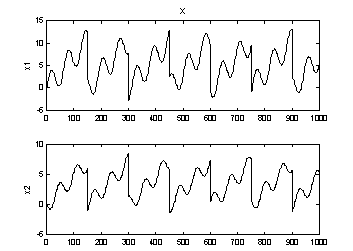Figure 2: Linearly transformed sources. The axes of the sources are drawn in the left plot.

## 3.2 Nongaussianity

Let yi be an estimated component (yi » si) and wi a row in the estimated inverse trans­formation matrix W » A-1. The reconstruction of the component is then yi = wi Tx.

Under certain conditions, the sum of independent random variables has a distribution that is closer to Gaussian than the distribution of the original variables . This theorem is the foundation for the ICA algorithm. It means that the distributions of x are more Gaussian that the distri­butions of s, because xi is a weighed sum of the components in s. As the components are known to be nongaussian and independent, the problem is now reduced to finding wi so that the nongaussianity of yi is maximized.

For that, some measure of nongaussianity is needed. One way of measuring nongaussianity is by the following approxi­mation to neg­entropy: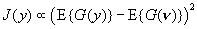(4)

µ denotes proportionality, but as we are only interested in the wi that maximizes J(wiTx), the actual value at the maximum is not important. n is a Gaussian variable with zero mean and unit variance, so the term E{G(n)} is a constant. G(y) is a non-quadratic function. The best choice of G(y) depends on the problem, but common used functions are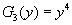(5)

with a1 Î [1; 2]. G3(y) gives a Kurtosis-based approximation. For G2(y), the constant term in (4) is given by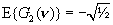.                                                             (6)

## 3.3 Preprocessing

Some preprocessing is useful before attempting to estimate W. First, the obtained signals should be centered by subtracting their mean value E{x}: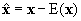(7)

Then they are whitened, which means they are linearly transformed so that the components are uncorrelated and has unit variance. Whitening can be performed via eigenvalue decom­position of the covariance matrix,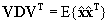. V is here the matrix of orthogonal eigen­vectors and D is a diagonal matrix with the corresponding eigenvalues. The whitening is done by multiplication with the transformation matrix P: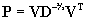(8)(9)

This is closely related to principal component analysis (PCA) .

The covariance of the whitened data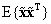equals the identity matrix, and the mixing matrix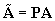is orthogonal (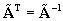). The matrix for extracting the independent components from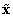is now denoted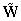, so.

Just to summarize the notation: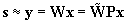(10)

Figure 3 is a (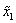,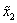) plot after centering and whitening of the random data from Figure 2 (left).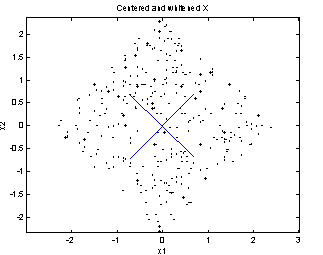Figure 3: Centered and whitened version of the random data from the example. The axes are now seen to be perpendicular.

## 3.4 The FastICA algorithm

We are ready to begin estimating wi which are now the rows in. As the variance of the independent components cannot be determined (limitation 1), it is natural to constrain the variance of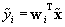to 1. When the data is whitened, this is equivalent to constraining the norm of wi to unity. In combination with (4), this gives an optimization problem:

Find the wi that maximizes J(wiT) under the constraint ||wi|| = 1                          (11)

Optimization of wi for the sine and triangular wave problem in Figure 2 (right) is illustrated in Figure 4. It is a contour plot of J(wT) with the axes w1 and  w2, the proportionality factor in (4) equal to one and G(y) = G2(y). The constraint is marked with a black circle with radius one. * indicates the maxima for w1 and w2, and because of the whitening they are orthogonal. Two maximas with the same nongaussianity exists for each component, namely at wi and –wi.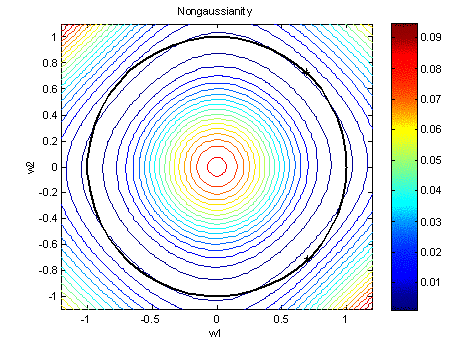Figure 4: Nongaussianity estimated by (4) with proportionality factor equal to one using G2(y). The optimization is constrained to the black circle and two maxima are marked with *.

From optimization theory we have that extrema of E{G(y)} are found where the gradient of the Lagrange function is zero . As the constraint ||w|| = 1 is equivalent to wTw–1 = 0, the Lagrange function is given by:

L(w,l) = E{G(wTx)} – l(wTw–1)                                                (12)

The gradient with respect to w is

Lw´(w,l) = E{xg(wTx)} – 2lw                                                  (13)

where g(y) is the gradient of G(y), so the solution is obtained where

E{xg(wTx)} – bw = 0,      b = 2l                                                (14)

The Lagrange multiplier b can be calculated to b = E{w*Txg(w*Tx)} where w* is the optimum .

The gradients of the G-functions in (5) are given by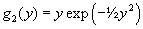(15)

With only two components, the problem can be reduced to one variable – the angular position on the circular constraint. That makes it possible to plot J(wT) and Lw´(w,l) as a function of the angle, which is done in Figure 5. ||Lw´(w,l)|| is plotted for the values of l evaluated with w* for both components. The figure demonstrates that the maxima for J(wT) are indeed found where Lw´(w,l) = 0.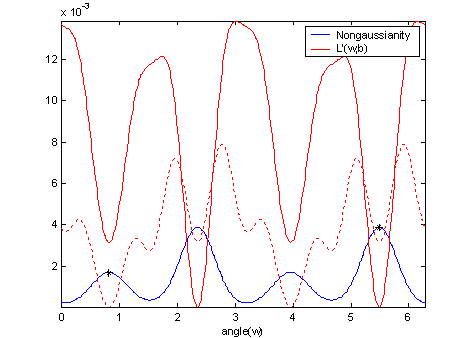Figure 5: Nongaussianity J(wT) using G2(y) and the gradient of the Lagrange function ||Lw´(w,l)|| is plotted for both components. Maxima for J(wT) are found where Lw´(w,l) = 0.

In the FastICA algorithm, the equation Lw´(w,l) = 0 is solved iteratively via Newton’s method. One solution for each component is wanted, so the optimization has to be run for one component at a time. The wi are orthogonal, so to prevent the same solution from being found more than once, a Gram-Schmidt-like decorrelation can be performed in each iteration. Some starting guess for wi is needed to initialize Newton’s method, and the order in which the components are found depend on this guess.

The algorithm should continue until some suitable stopping criteria are satisfied. Typically this would be that either wi is converged to the solution or too many iterations has been performed. Convergence can be checked by comparing wi to that from the previous iteration: If their dot product is close to one, they are almost equal which indicates convergence. This gives the following algorithm, which should be run for i = 1...number of components:

Initialize wi to starting guess

While not converged:// Newton step wi :=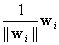// Normalization wi := wi –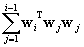// Decorrelation wi :=// Normalization

(16)

When the algorithm has finished,is estimated and the components can be reconstructed using (10). The result of applying the method to the example problem is shown in Figure 6. On this simple problem, the reconstruction is almost perfect. The estimate of the mixing matrix is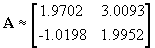(17)

In this example, both sign and order of the components came out correct, but they could just as well be swapped as described in the limitations. The energy (variance) is correct because the original sources had unit variance.Figure 6: ICA reconstruction of the mixed signals from Figure 2 (right).

## 3.5 Implementation

A Matlab implementation of the method is shown in Appendix 1. A short description of how the program is used is included, and the source has comments describing each code section. Except for a small variation in the FastICA algorithm, it is implemented as described in the previous sections. As starting guess foris used the unit matrix.

For a real data sequence, only estimates of the mean E{x} and covariance matrix E{xxT} can be computed. Therefore only an estimate ofcan be found, and in some cases better results can be achieved by not forcing it to be exactly orthogonal as the whitening is not perfect. A zoom on Figure 5 around the optimum for the second component is included in Figure 7. It shows that the value returned by algorithm (16) is not the in the maximum of the nongaussianity function when the wi are forced to be orthogonal.

The purpose of the Gram-Schmidt decorrelation/orthogonalization performed in the algorithm is to avoid finding the same component more than on once. When close to a zero, Newton’s method will usually keep converging towards that zero, so by turning off the decorrelation when close to a zero, the orthogonality constraint is loosened.

In the implementation this is done by dividing the optimization into two steps: First algorithm (16) is ran until convergence. That should get us close to the maximum. Then iterations per­form­ing only the Newton step and normalization are done until convergence. With this modification the true maximum is found, see Figure 8.

A parameter to the Matlab function decides whether decorrelation is performed all the way or not. For long data sequences, the estimates of E{x} and E{xxT} are usually better, and it is not necessary to divide the optimization into two steps. It can also be specified that if decorrelation is stopped and the same component is found twice, then the orthogonalized version should be used.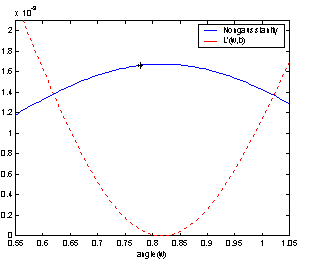Figure 7: Because of the orthogonalization, the optimum found might not be the maximum of the nongaussianity function.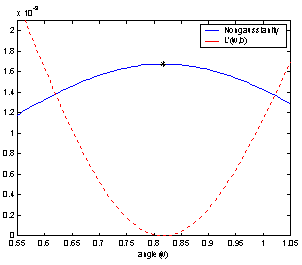Figure 8: When the orthogonalization is stopped close to the optimum, the Newton steps will find the zero.

Another example with four sources of artificial data is shown in Figure 9 through Figure 12. The mixing matrix used is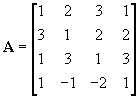(18)

With that many sources it is almost impossible to guess the shape of the waves by just looking at the mixtures. But the ICA result is very good, especially when decorrelation is stopped close to the optimum. MSE of matching sources/components was computed to compare the results, and not forcing orthogonality reduced the MSE to less than half. Which of the tree G-functions are used for estimating nongaussianity does not seem to be important, but G3(y) results in a little less MSE that the other two, so that is chosen for the problem.

The estimated transformation matrix used for Figure 12 is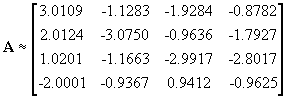.                                      (19)

In this example neither the order nor the sign of the sources has been preserved, meaning that some columns are swapped and/or has changed sign.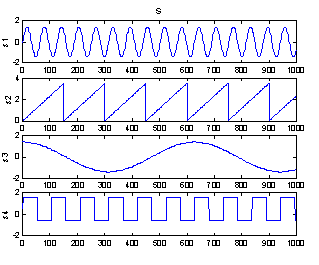Figure 9: Sources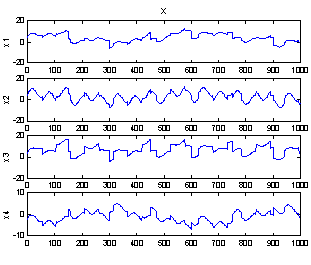Figure 10: Mixed signalsFigure 11: Result of ICA with forced ortho­go­nal­ization.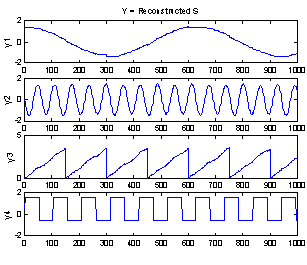Figure 12: Result of ICA with ortho­go­nal­ization stopped close to optimum.

# 4 Application

The recordings available here only have seven EEG channels plus an EOG channel. Modern equipment usually has more channels – up to 256. Less than 32 is usually considered too few for practical use of ICA on EEG data, but the following examples do show some capabilities.

In section 3.1 some assumptions made in deriving the ICA model were listed. Not all of them are completely satisfied for EEG data: Because of the many connections in the neural network in our brain, there is some dependence between the sources. It is also very unlikely that the number of sources match the number of electrodes. Volume conduction in brain tissue is effectively instantaneously and does produce a linear combination of the sources, so assumptions 3 and 4 are satisfied .

## 4.1 ICA for evoked potential source separation

From the 120 epochs recorded from each subject, the response evoked by the click sound is extracted. Some epochs containing EMG noise forcing the A/D converter to saturation were removed, and epochs with EOG activity greater than 70mV were also not used. The EP is extracted by averaging of the remaining epochs. ICA is then applied to the 500 samples extracted in the first 500 ms after stimulus onset.

To illustrate how the independent components are computed from the electrode signals, a little head with the weights are shown for each component. For the i’th components, the weights correspond to the elements in the i’th row in W. The values shown are in % and normalized so the sum of absolute weight values equal 100%. The weights are shown on a head like Figure 13 with the seven electrodes in the order Fp1, Fp2, Fz, Cz, C3', C4' and Pz.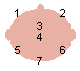Figure 13: Positions of the seven electrodes. Note that this is not the exact electrode placement, just a simplified illustration.

Because order and sign of the components extracted is random, they are reordered in the following figures so that similar components are shown in the same position and with same sign every time. This is just done to make visual comparison of the results easier.

ICA of the EEG data was done using the Matlab implementation in Appendix 1. If decor­relation of the wi vectors was stopped close to the optimum, it sometimes happened with this data set that the same component was found twice. Thereforewas forces orthogonal. Stopping criteria for the optimization: Dot product of wi from two consecutive iterations less that 10-6 from 1, or number of iteration steps exceeded 100. This is achieved by using the default options for ica.m: opts parameter set to [100, 10e-6, inf].

Figure 14 shows the evoked potential extracted from the seven channels. It is clear that these mixed signals are highly correlated. In Figure 15 are the seven components found via ICA and their weights from the W matrix. G(y) in the nongaussianity estimate (4) is chosen to G2(y). As the relative energy of the original sources cannot be determined (limitation 1), the weight values are not comparable between the components.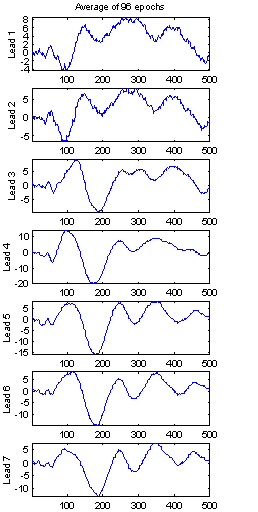14: EP extracted from the recording from subject 103.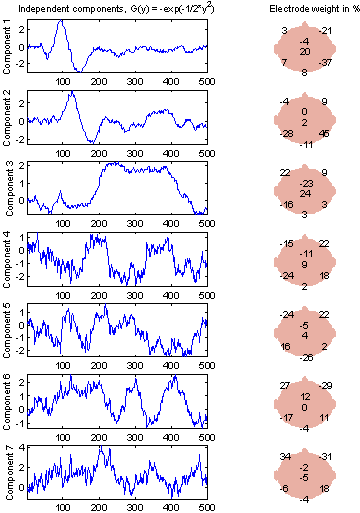Figure 15: Independent components in the EP from subject 103. Decorrelation is used in all FastICA iterations. G(y) = G2(y).

Interpretation of the components is hard without further studies. It is probable that some of the components are related to sources generating the EP, and some are related to background activity. Component 1 seems to be responsible for the P50 and N100 peaks (with sign inverted), perhaps in combination with component 2. The last four components have more high-frequency contents.

Figure 16 and Figure 17 show the components extracted using the other two G-functions. They are very similar to Figure 15, especially G1(y), which indicates that choice of G-function is not important. The components with G3(y) used does seem to be more noisy, so from here only G2(y) is used.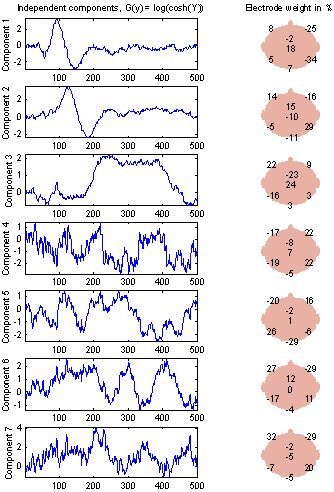16: ICA with G(y) = G1(y), a1 = 1.17: ICA with G(y) = G3(y).

The components found for EPs extracted from different subjects are not the same. An example from another subject is found below. The extracted EP in Figure 18 is also very different from that in Figure 14, so it is not surprising that other components are found. In Figure 19 N100 and maybe P50 still seems to come from the first two components (again with sign reversed), but the remaining components are hard to compare.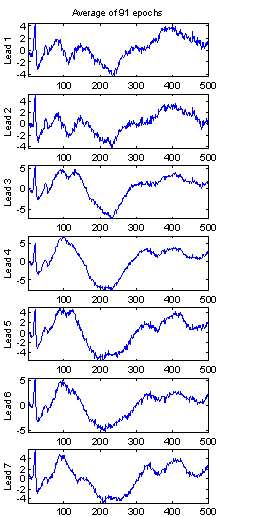Figure 18: EP extracted from the recording from subject 102.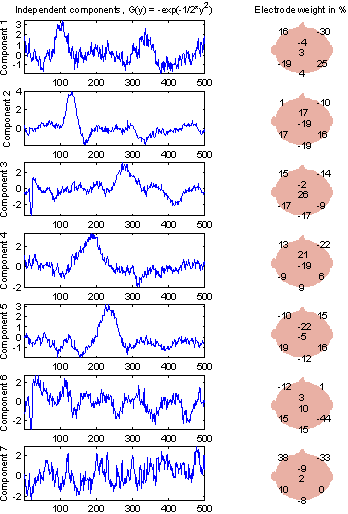Figure 19: Independent components in the EP from subject 102. G(y) = G2(y).

## 4.2 ICA for artifact reduction

Eye movement, blinks or other EMG artifact in the epochs will reduce the quality of the evoked potential extraction. For extracting the EPs used in section 4.1, plenty of data was available so epochs with such artifacts could just be rejected. That is not always the case, so methods for reducing the artifacts are sought after. Jung et al. (1998) performs ICA of the raw EEG and demonstrates that the unwanted artifact will be contained in one component. If the signals at the electrodes are wanted without the artifact, that component can be removed and the signals reconstructed from the remaining components.

The output from the ICA algorithm was the W matrix. The components are computed as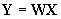, where X is a matrix with the samples from one electrode signal in each row. If the i’th row in Y is the artifact component, it can be removed from the recording by computing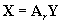, where Ar is the inverse of W with the i’th column replaced by zeros.

Figure 20 on the next page is an example of applying the method. ICA is done with G2(y).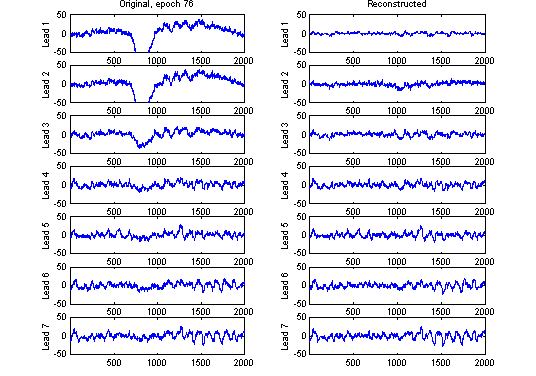20

A two-second clip from before the event in one of the epochs was used. It is non-averaged data, so little structure is visible except for some alpha activity. As expected, the eye movement primarily affects the two frontal channels, but also the third (Fz) has significant artifact. In the reconstruction after removing component 1 almost no trace of the movement is visible, and the structure of the signals seems to be preserved. In this example the artifact is only visible in the first independent component, which explains the good result.

Another example where some artifact is seen in all obtained signals is shown in Figure 21. The artifact is not completely contained in one component, so even though the reconstruction is much better than the original, it is not fully cleaned.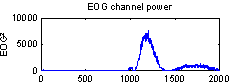Figure 21: Another example of artifact reduction.

Which component contains the artifact can vary, but it is easy to create an algorithm that auto­matically removes it when the EOG channel is available: The results presented here are produced by removing the component with the largest correlation coefficient (absolute value) when compared to the EOG channel. The sign and energy of the components therefore have no influence on which component is chosen, so the limitations to ICA is not a problem in this method. It has been tested on many recordings, and the results produced were similar to those shown in the two examples.

# 5 Conclusion

Independent component analysis was described as a new tool for digital signal processing and applied to analyzing EEG data. It was introduced including the assumptions made and the limitations of the results. A recently developed effective implementation of ICA based on a general method for constrained optimization was presented.

The method performed very well on example problem with artificial data, and the mean square error between the output and the true sources was small. Used on evoked potentials extracted from EEG recordings, it is able to find some components that make up the EP and some probably related to noise in the data, but they are hard to interpret without further studies.

The most popular implementation of ICA today (measured by ranking on the global Internet search engine Google ), Gävert et al. (2001), only performed slightly better than the Matlab imple­men­tation given here on the artificial data. On the EEG data, it failed to separate components in some cases, so it has not been used in the examples. The comparison to the published program indicates that the included implementation can be of practical use.

An automatic algorithm for removing artifacts caused by eye movement was suggested based on a previous study of artifact rejection. The method performed well on the available seven-channel data set and the artifacts was considerably reduced. Only visual evaluation of the results was performed however, so final conclusions on the value of the method requires tests in a specific application.

# 6 Literature

Gävert, Hugo; Hurri, Jarmo; Särelä, Jaakko and Hyvärinen, Aapo

FastICA for Matlab 5.x, version 2.1, 2001-1-15

Matlab implementation of the FastICA algorithm available from

http://www.cis.hut.fi/projects/ica/fastica/

Hyvärinen, Aapo

The Fixed-Point Algorithm and Maximum Likelihood Estimation for Independent Component Analysis

Laboratory of Computer and Information Science, Helsinki University of Technology

(not dated)

http://www.cis.hut.fi/~aapo/

Hyvärinen, Aapo and Oja, Erkki

Independent Component Analysis: A Tutorial

Laboratory of Computer and Information Science, Helsinki University of Technology

1999

http://www.cis.hut.fi/~aapo/

Højen-Sørensen, Pedro A.d.F.R.; Winther, Ole and Hansen, Lars Kai

Mean Field Approaches to Independent Component Analysis

IMM, DTU, 2001-1-26

Jung, Tzyy-Ping;  Humphries, Colin;  Lee, Te-Won;  Makeig, Scott;  McKeown, Martin J.;  Iragui, Vincent and  Sejnowski, Terrence J.

Removing electroencephalographic artifacts: comparison between ICA and PCA

Neural Networks for Signal Processing VIII, 1998

Madsen, Kaj; Nielsen, Hans Bruun and Tingleff, Ole

Optimization with Constraints

IMM, DTU, 2001-12-10

http://www.imm.dtu.dk/courses/02611/

Makeig, Scott;  Bell, Anthony J.;  Tzyy-Ping Jung  and Sejnowski, Terrence J.

Independent component analysis of electroencephalographic data

Advances in Neural Information Processing Systems 8

MIT Press, 1996

Makeig, Scott;  Jung, Tzyy-Ping;  Bell, Anthony J.;  Ghahremani, Dara and Sejnowski, Terrence

Blind separation of auditory event-related brain responses into independent components

Proceedings of the National Academy of Sciences of the USA

Issue Vol.94 Issue.20, 1997

Pope, K.J. and Bogner, R.E.

Blind Signal Separation I: Linear, Instantaneous Combinations

Cooperative Research Center for Sensor Signal and Information Processing, SPRI

Digital Signal Processing 6, 5-15, 1996

Pope, K.J. and Bogner, R.E.

Blind Signal Separation II: Linear, Convolutive Combinations

Cooperative Research Center for Sensor Signal and Information Processing, SPRI

Digital Signal Processing 6, 17-28, 1996

Vinther, Michael

AEP analysis in EEG from schizophrenic patients using PCA

Ørsted, DTU, 2002-6-7

http://logicnet.dk/reports/

%Independent component analysis

%  [Y,W,P] = ica(X,G,opts)

%

%Input parameters

%  X    : Matrix with original components in the columns.

%  G    : Nonlinearity used in nongaussianity estimate: (optional)

%           'kurt'   G(y) = y^4

%           'cosh'   G(y) = log(cosh(y))

%           'gauss'  G(y) = -exp(-1/2*y^2)     (default)

%  opts : Vector with three elements. The first two are used in stopping

%         criteria for each component: (optional)

%           Number of iterations>opts(1) or

%           1-abs(w'*wOld)<opts(2). (Dot product close to 1).

%         opts(3) decides how decorrelation is performed. If the difference

%         (one-norm) between two rows in W is less than or equal to opts(3),

%         one of them is replaced by a decorrelated version. Use opts(3)=inf

%         to decorrelate after all iterations. Decorrelation gives less

%         nongaussianity, but prevent two components from being equal.

%         Default is opts = [100,10e-6,1]

%

%Output parameters

%  Y : Matrix with independent components in the columns.

%  W : Transformation matrix so that Y = (W*X')'

%  P : Convergence. Sum of abs(w'*wOld) for all components in all iterations.

%

%2002-12-04 | Michael Vinther | mv@logicnet.dk

function [Y,W,P] = ica(X,G,opts)

if nargin<2

G = 'gauss';

end

if nargin<3

opts = [100,10e-6,1];

end

% Centering

m = mean(X)';

Xc = X'-repmat(m,1,size(X,1)); % Center and transpose

% Whitening

R = cov(Xc');

[V,D] = eig(R);

WhiteT = V*diag(diag(D).^-0.5)*V'; % Whitening transform

Xw = WhiteT*Xc;

% Select G

switch lower(G)

case 'kurt'

g = inline('x.^3'); gg = inline('3*x.^2');

case 'cosh'

g = inline('tanh(x)'); gg = inline('1-tanh(x).^2');

case 'gauss'

g = inline('x.*exp(-0.5*x.^2)');

gg = inline('exp(-0.5*x.^2)-x.^2.*exp(-0.5*x.^2)');

otherwise

error('Illegal value for G');

end

% Estimate W by FastICA

if nargout>2

[Ww,P] = fastica1(Xw,opts,g,gg);

else

Ww = fastica1(Xw,opts,g,gg);

end

% Reconstruct

W = Ww*WhiteT;

Y = (W*X')';

Support function for ica.m. fastica1 is an implementation of algorithm (16) with the possibility of turning off decorrelation close to the optimum.

%FastICA algorithm

%  [W,P] = fastica1(X,opts,g,gg)

%

%Input parameters

%  X    : Matrix with components in the columns. Components are assumed to be

%         uncorrelated and have zero mean (whitened and centered).

%  opts : Vector with three elements. The first two are used in stopping

%         criteria for each component:

%           Number of iterations>opts(1) or

%           1-abs(w'*wOld)<opts(2). (Dot product close to 1).

%         opts(3) decides how decorrelation is performed. If the difference

%         (one-norm) between two rows in W is less than or equal to opts(3),

%         one of them is replaced by a decorrelated version. Use opts(3)=inf

%         to decorrelate after all iterations. Decorrelation gives less

%         nongaussianity, but prevent two components from being equal.

%  g    : First derivative of nongaussian estimator.

%  gg   : Second derivative of nongaussian estimator.

%

%Output parameters

%  W    : Inverse transformation matrix.

%  P    : Convergence. Sum of abs(w'*wOld) for all components in all

%         iterations.

%

%2002-11-21 | Michael Vinther | mv@logicnet.dk

function [W,P] = FastICA1(X,opts,g,gg)

nComp = size(X,1);

W = eye(nComp); % Initial guess on W

P = [];

sumP = 0;

wDecor = [];

for c=1:nComp % For all components

wOld = zeros(nComp,0);

w = W(c,:)';

i = 0;

decorrelate = c>1; % Decortrelate all but first component

stop = 0;

while ~stop

wOld = w;

i = i+1;

% Do Newton-Rhapson step

y = w'*X;

w = mean((X.*repmat(g(y),nComp,1))')'-mean(gg(y))*w;

w = 1/norm(w)*w;

if decorrelate % Decorrelate w

wd = zeros(nComp,1);

for d=1:c-1

wd = wd+w'*W(d,:)'*W(d,:)';

end

w = w-wd;

w = 1/norm(w)*w;

end

if nargout>1

P(end+1) = sumP+abs(w'*wOld);

end

if 1-abs(w'*wOld)<opts(2) % No change in w

if decorrelate & opts(3)<inf

decorrelate = 0; % Stop decorrelation

wDecor = w;

else

stop = 2;

end

elseif i>=opts(1) % Too many iterations

stop = 1;

end

end

if ~isempty(wDecor) % Check if w already found

for d=1:c-1

Diff(d) = min(norm(W(d,:)'-w,1),norm(W(d,:)'+w,1));

end

if min(Diff)<=opts(3)

w = wDecor; % Close match found, use decorrelated w

end

end

W(c,:) = w';

if nargout>1

sumP = P(end);

end

end

 The Central Limit Theorem, Hyvärinen and Oja (1999) section 4.1.

 Eigenvalue decomposition and PCA is described in detail in Vinther (2002).

 The Kuhn-Tucker condition, Madsen et. al (2001) theorem 2.9.

 The value of b and details of solving the problem via Newton’s method is given in Hyvärinen (not dated).

 Discussion of which assumptions are satisfied can be found in Makeig et al. (1996) and Jung et al. (1998).